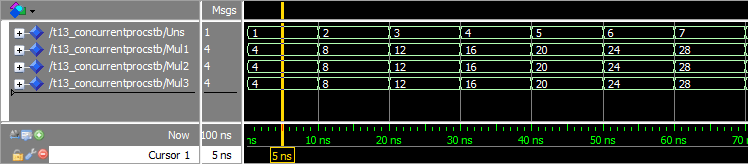# HOW TO CREATE A CONCURRENT STATEMENT IN VHDL

A concurrent statement in VHDL is a signal assignment within the architecture, but outside of a normal process construct. The concurrent statement is also referred to as a concurrent assignment or concurrent process.

When you create a concurrent statement, you are actually creating a process with certain, clearly defined characteristics. Concurrent statements are always equivalent to a process using a sensitivity list, where all the signals to the right of the signal assignment operator are on the sensitivity list.

These shorthand notation processes are useful when you want to create simple logic which results in the assignment of a single signal. Instead of typing out a full process construct with sensitivity lists and all of that, you can simply assign to the target signal directly in the architecture.

When used correctly, the intention of the code will still be pretty clear. No need to create a process for every single bit you want to flip.

library ieee;

use ieee.std_logic_1164.all;

use ieee.numeric_std.all;

entity T13_ConcurrentProcsTb is

end entity;

architecture sim of T13_ConcurrentProcsTb is

signal Uns :  unsigned(5 downto 0) := (others => '0');

signal Mul1 : unsigned(7 downto 0);

signal Mul2 : unsigned(7 downto 0);

signal Mul3 : unsigned(7 downto 0);

begin

process is

begin

Uns <= Uns + 1;

wait for 10 ns;

end process;

-- Process multiplying Uns by 4

process is

begin

Mul1 <= Uns & "00";

wait on Uns;

end process;

-- Equivalent process using sensitivity list

process(Uns) is

begin

Mul2 <= Uns & "00";

end process;

-- Equivalent process using a concurrent statement

Mul3 <= Uns & "00";

end architecture;We can see from the waveform that Mul1, Mul2, and Mul3 behave exactly the same. This is because the concurrent statement and the two processes we created are equivalent.

A concurrent statement works just like a process. All signals to the right of the <= are automatically added to the sensitivity list. This means that the signal to the left of the <= will be updated whenever one of the signals that are evaluated change.

There are many ways to multiply numbers in VHDL. In this exercise we multiplied the Uns signal by 4, using bit shifting. All our signals are of unsigned type, meaning that they are interpreted by numbers. Appending a 0 to the right of a binary number is the same as multiplying it by 2.

This is an illustration of what happens at the cursor in the waveform:• A concurrent statement is a signal assignment directly in the architecture region
• Concurrent statements are equivalent to a process with all evaluated signals on the sensitivity list

با & کردن 00 عمل ضرب در 4 انجام میشود.

`library` `ieee``;`
`use` `ieee``.``std_logic_1164``.``all``;`
`use` `ieee``.``numeric_std``.``all``;`

`entity` `T13_ConcurrentProcsTb ``is`
`end` `entity``;`

`architecture` `sim ``of` `T13_ConcurrentProcsTb ``is`

`    ``signal` `Uns :  ``unsigned``(5 ``downto` `0) := (``others` `=> ``'0'``);`
`    ``signal` `Mul1 : ``unsigned``(7 ``downto` `0);`
`    ``signal` `Mul2 : ``unsigned``(7 ``downto` `0);`
`    ``signal` `Mul3 : ``unsigned``(7 ``downto` `0);`

`begin`

`    ``process` `is`
`    ``begin`

`        ``Uns <= Uns + 1;`

`        ``wait` `for` `10 ns;`
`    ``end` `process``;`

`    ``-- Process multiplying Uns by 4`
`    ``process` `is`
`    ``begin`

`        ``Mul1 <= Uns & ``"00"``;`

`        ``wait` `on` `Uns;`

`    ``end` `process``;`

`    ``-- Equivalent process using sensitivity list`
`    ``process``(Uns) ``is`
`    ``begin`

`        ``Mul2 <= Uns & ``"00"``;`

`    ``end` `process``;`

`    ``-- Equivalent process using a concurrent statement`
`    ``Mul3 <= Uns & ``"00"``;`

`end` `architecture``;`

## تبلیغات